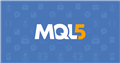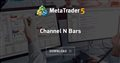# Printing a line at the lowest low of the last 100 candles

Hello guys,

can someone tell my why this code doesn't work correctly?

```#property indicator_chart_window
#property indicator_buffers 1
#property indicator_plots   1

#property indicator_type1   DRAW_LINE
#property indicator_color1  clrBlack
#property indicator_width1  1

double buf[];
//+------------------------------------------------------------------+
//| Custom indicator initialization function                         |
//+------------------------------------------------------------------+
int OnInit() {

SetIndexBuffer(0,buf,INDICATOR_DATA);
PlotIndexSetDouble(0,PLOT_EMPTY_VALUE,0);

//---
return(INIT_SUCCEEDED);
}
//+------------------------------------------------------------------+
//| Custom indicator iteration function                              |
//+------------------------------------------------------------------+
int OnCalculate(const int rates_total,
const int prev_calculated,
const datetime &time[],
const double &open[],
const double &high[],
const double &low[],
const double &close[],
const long &tick_volume[],
const long &volume[],
{
//---

int limit=prev_calculated-1;
if (prev_calculated==0) limit=0;

for (int i=limit;i<rates_total;i++) {
buf[i]=low[iLowest(_Symbol,PERIOD_CURRENT,MODE_LOW,100,i)];
}
//--- return value of prev_calculated for next call
return(rates_total);
}```

You don't need to use 'iLowest' - you already have access to the ' low ' array in OnCalculate. You must use the ' ArrayMinimum ' function.

```//+------------------------------------------------------------------+
//|                                                         Test.mq5 |
//|                                  Copyright 2022, MetaQuotes Ltd. |
//|                                             https://www.mql5.com |
//+------------------------------------------------------------------+
#property version   "1.00"
#property indicator_chart_window
#property indicator_buffers 1
#property indicator_plots   1
//--- plot Lowest
#property indicator_label1  "Lowest"
#property indicator_type1   DRAW_LINE
#property indicator_color1  clrYellowGreen
#property indicator_style1  STYLE_SOLID
#property indicator_width1  1
//--- input parameters
input int      Input1=9;
//--- indicator buffers
double         LowestBuffer[];
//+------------------------------------------------------------------+
//| Custom indicator initialization function                         |
//+------------------------------------------------------------------+
int OnInit()
{
//--- indicator buffers mapping
SetIndexBuffer(0,LowestBuffer,INDICATOR_DATA);
//--- set accuracy
IndicatorSetInteger(INDICATOR_DIGITS,Digits());
//---
return(INIT_SUCCEEDED);
}
//+------------------------------------------------------------------+
//| Custom indicator iteration function                              |
//+------------------------------------------------------------------+
int OnCalculate(const int rates_total,
const int prev_calculated,
const datetime &time[],
const double &open[],
const double &high[],
const double &low[],
const double &close[],
const long &tick_volume[],
const long &volume[],
{
if(rates_total<Input1)
return(0);
//---
int limit=prev_calculated-1;
if(prev_calculated==0)
limit=Input1;
for(int i=limit; i<rates_total; i++)
{
LowestBuffer[i]=low[ArrayMinimum(low,i-Input1,Input1)];
}
//--- return value of prev_calculated for next call
return(rates_total);
}
//+------------------------------------------------------------------+
```Documentation on MQL5: Array Functions / ArrayMinimum
• www.mql5.com
ArrayMinimum - Array Functions - MQL5 Reference - Reference on algorithmic/automated trading language for MetaTrader 5
Files:
Test.mq5  3 kb

There is a small error in my code - but this is a task for attentiveness :)

There is a small error in my code - but this is a task for attentiveness :)

I saw the small error and corrected it. :)

Thank you very much for your help. The indicator works perfect now!

Marbo # :

I saw the small error and corrected it. :)

Thank you very much for your help. The indicator works perfect now!

You can see the channel ( Channel N Bars ) which is built either by High Low or Close Open.Channel N Bars
• www.mql5.com
Channel of 'N' bars at 'Low' and 'High' prices
Reason: